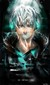frey443Offline
Neophyte
Joined: 2017/11/19

I've been working whenever I can, on a Tor Address Indexer in C and possibly in Python or Perl.

The idea is simple enough:

1) Generate a random Tor address string using rand() and math... (yes guys this involves math because of the algorithm)
2) "Ping" the host to see if it is "alive" and return data (issue is that tor address don't have dns leaks)
3) If "alive", save the name and address to a db

Simpler said than done.

I've coded a random generator using C, but the issue is the algorithm because of how the addresses are generated and also how rand() works.

My code is:

#include <stdio.h>
#include <stdlib.h>
#include <time.h>

//compile: gcc tor_generator.c -l pthread -o tor_generator

void *generator()
{
int i;
char random_char;

const unsigned int min = 122;
const unsigned int max = 48;
const unsigned int range = max + min;
const unsigned int filter_min = 58;
const unsigned int filter_max = 96;

//seed
srand(time(0));

while(1)
{
for(i = 0; i <= 15; i++)
{

random_char = rand() % range;

if(random_char >= filter_min && random_char <= filter_max)
{
puts("[!] Invalid value found!");
break;

}
else if(i == 15 && random_char < filter_min && random_char > filter_max)
{
puts(random_char);

break;
exit(1);
}

}
}

return 0;

}

int main()
{

int i;

//for loop to generate the threads
for(i = 0; i < 10; i++)